# Abstract

This work evaluates proposed methods to correct engine power output as a function of atmospheric conditions. The analysis was made through experiments carried out in a vehicle on the road, under different temperature, pressure and air humidity conditions. The vehicle had a four-cylinder gasoline-fuelled engine, with multi-point fuel injection system, variable intake pipe length and variable intake valve camshaft position. The vehicle was tested at sea level and at 827 m above sea level, corresponding to atmospheric pressures between 1027 and 926 mbar. Air temperature varied from 22,8 to 33,8 °C at the test locations. The measured performance parameter in the tests was the vehicle acceleration time. The acceleration times from 0 to 400 m, 0 to 1000 m, 40 to 100 km/h and 80 to 120 km/h were all recorded, leaving from an initial vehicle speed of 40 km/h. The engine power curve obtained in laboratory under a standard ambient condition was corrected to the conditions of the road tests by the correction factors proposed by the methods under evaluation, and the corresponding acceleration times were calculated and compared with the measurements from the road tests. The evaluated methods for power correction were the following: DIN 70020, SAE J 1349, JIS D 1001 and ISO 1585. The SAE J 1349 method provided the best approach between the experimental and calculated acceleration times.

Internal combustion engine; acceleration time; atmospheric pressure; temperature; power

TECHNICAL PAPERS

Comparison of engine power correction factors for varying atmospheric conditions

J. R. SodréI; S. M. C. SoaresII

IPontifícia Universidade Católica de Minas Gerais, Programa de Engenharia Mecânica, Av. Dom José Gaspar, 500 - Coração Eucarístico, 30535-610 Belo Horizonte,- MG. Brazil, ricardo@pucminas.br IIFIAT Automóveis S.A. , Divisão de Experimentação de Veículos, Rodovia Fernão Dias, BR 381, km 429, 32530-000 Betim, MG. Brazil, sandro.soares@fiat.com.br

ABSTRACT

This work evaluates proposed methods to correct engine power output as a function of atmospheric conditions. The analysis was made through experiments carried out in a vehicle on the road, under different temperature, pressure and air humidity conditions. The vehicle had a four-cylinder gasoline-fuelled engine, with multi-point fuel injection system, variable intake pipe length and variable intake valve camshaft position. The vehicle was tested at sea level and at 827 m above sea level, corresponding to atmospheric pressures between 1027 and 926 mbar. Air temperature varied from 22,8 to 33,8 °C at the test locations. The measured performance parameter in the tests was the vehicle acceleration time. The acceleration times from 0 to 400 m, 0 to 1000 m, 40 to 100 km/h and 80 to 120 km/h were all recorded, leaving from an initial vehicle speed of 40 km/h. The engine power curve obtained in laboratory under a standard ambient condition was corrected to the conditions of the road tests by the correction factors proposed by the methods under evaluation, and the corresponding acceleration times were calculated and compared with the measurements from the road tests. The evaluated methods for power correction were the following: DIN 70020, SAE J 1349, JIS D 1001 and ISO 1585. The SAE J 1349 method provided the best approach between the experimental and calculated acceleration times.

Keywords: Internal combustion engine, acceleration time, atmospheric pressure, temperature, power

Introduction

The atmospheric conditions are known to affect the performance of internal combustion engines. A different engine performance from that specified by the manufacturer can be observed when the engine is working under an atmospheric condition different from that at which the engine data was collected by the manufacturer. The influence of the atmospheric condition on the performance of an engine can especially be observed through variations on the vehicle acceleration time and on fuel consumption. Correction factors are available to predict the power developed by the engine under distinct ambient conditions from the engine power curve obtained in laboratory tests at a standard condition. However, the many correction factors proposed often lead to different results for a same atmospheric condition considered. These non uniform results from correction factors motivates this investigation on which one better predicts the engine performance on the road.

Many works which are dedicated to verify the influence of the atmospheric parameters on the performance of an engine are referred to aviation applications. In those situations, high variations on altitude influence the engine power output, due to variations on air temperature, pressure and humidity. The works dedicated to the automotive industry usually describe experiments on a dynamometer bench, where each atmospheric parameter is varied one at a turn. This technique leads to a better understanding on the influence of an individual parameter, but do not give information on the simultaneous variation of the atmospheric parameters as in real conditions on the road. Thus, the objective of this work is to compare the results from different methods proposed to correct the power developed by the engine under varying atmospheric conditions. Experiments were carried out with a vehicle on the road under different conditions of pressure, temperature and humidity, where the engine performance was monitored through measurements of the vehicle acceleration time. The correction factors were used to correct the engine power curve available from laboratory tests at a standard ambient condition to the conditions of the road tests. The acceleration time calculated from the corrected power was compared to the vehicle acceleration time measured on the road. The correction factors evaluated are those proposed from DIN 70020 (DIN, 1986), SAE J 1349 (SAE, 1995), JIS D 1001 (JIS, 1993) and ISO 1585 (ISO, 1992; ABNT, 1996).

Nomenclature

a = vehicle acceleration (m/s2)

A fv= frontal vehicle area (m2)

b1, b2= coefficients defined by Eqs. (13) and (14) (dimensionless)

Cr = rolling resistance coefficient (dimensionless)

Cx = aerodynamic drag coefficient (dimensionless)

CF1...CF4= correction factors defined in Eqs. (1) to (4) (dimensionless)

d = vehicle position (m)

g = free fall acceleration (m/s2)

Ie = engine moment of inertia (N.m2)

Iw= wheel moment of inertia (N.m2)

Kr= rolling coefficient (dimensionless)

mv= vehicle mass (kg)

M = molecular weight of atmospheric air-water vapor mixture (kg/kmol)

N = rotational engine speed (rev/s)

p = atmospheric pressure (bar)

p bt = back tire pressure (bar)

p ft= front tire pressure (bar)

pv= water vapor partial pressure (bar)

P = output power (W)

Pa = power available to accelerate the vehicle (W)

Pd = power to overcome aerodynamic drag (W)

Pe = engine output power (W)

Pr = power to overcome rolling resistance (W)

rd= differential gear ratio (dimensionless)

rg= intermediate gear ratio (dimensionless)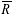= universal gas constant (=8314 J/kmol.K)

Ra=- drag force or aerodynamic resistance (N)

Rt= tire radius (m)

t = time (s)

T = ambient air temperature (K)

n = vehicle speed (m/s)

W bs= back shaft load (N)

W fs= front shaft load (N)

Wv = total vehicle weight (N)

Greek Letters

Dt= time interval (s)

f = air relative humidity (dimensionless)

r = air density (kg/m3)

Subscripts

0 =- reference condition

i = initial

f = final

Literature Survey

Higher atmospheric pressures increase the air density and, therefore, augment the intake air charge to the engine. A higher intake charge improves the engine volumetric efficiency, and more power is delivered (Pulkrabek, 1997; Heywood, 1988; Giacosa, 1986). From an extensive laboratory work on a gasoline engine, Nakajima et a. (1969) concluded that brake torque and specific fuel consumption vary directly proportional to atmospheric pressure.

The ambient temperature is found to affect the flame speed, the combustion reaction rate, the uniformity of the fuel-air mixture, the volumetric efficiency and the heat transfer rate though the cylinder walls (Pulkrabek, 1997). Higher intake air temperatures increase the occurrence of engine knock and decrease the volumetric efficiency (Heywood, 1988). Higher intake air temperatures can also contribute to stratified evaporation of the fuel components in the chamber. Each fuel component evaporates at a specific temperature, causing cycle-to-cycle combustion variations (Giacosa, 1986). On the other hand, for lower temperatures, only a small part of the injected fuel is vaporized, causing non-homogeneity. As a result, lower flame speeds, higher unburned mixture, higher hydrocarbons and carbon monoxide emissions, and loss of power are observed (Pulkrabek, 1997; Heywood, 1988; Giacosa, 1986).

Pre-heated intake mixture at low rotational speed improves combustion. At high engine rotational speeds, higher intake mixture temperatures decrease the volumetric efficiency of the engine (Chiu and Horng, 1992). Nakajima et al. (1969) found that engine brake torque, specific fuel consumption and volumetric efficiency vary inversely proportional to the square root of the suction air temperature.

Air humidity influences the performance of an engine, though not comparable to the influence of pressure and temperature (Redsell et al., 1993). High air humidity decreases the thermal efficiency and, thus, reduces the engine mean effective pressure (Taylor, 1992). Also, increasing air humidity diminishes the flame speed, slowing down combustion (Giacosa, 1986). High air humidity is beneficial to the engine at high cylinder temperatures, as it prevents the occurrence of knocking by heat absorption during the dissociation process of water molecules (Giacosa, 1986). The presence of water in the intake air can also reduce oxides of nitrogen and carbon monoxide emissions (Tsao and Miller, 1984).

Data on the influence of the atmospheric pressure, temperature and humidity on the acceleration time of a vehicle were obtained by Soares and Sodré (2002). The authors carried out road tests under varying atmospheric conditions, and have shown that the atmospheric pressure have a higher influence on the acceleration time than the air temperature. The results from the road tests performed by the authors are used in this work to evaluate power correction factors, as described in the following sections.

To correlate the engine performance measured in dynamometer tests to the engine performance at different ambient conditions, correction factors for the engine power delivery are proposed by institutions, such as DIN (Deutsches Institut für Normung), SAE (Society of Automotive Engineers), ISO (International Organization for Standardization), JIS (Japanese Industrial Standard) and ABNT (Brazilian Association of Technical Standards). These correction factors can be used to estimate the main performance parameters, such as power, torque, mean effective pressure and acceleration time, from available laboratory data to different atmospheric conditions.

Experiments

Equipment and Instrumentation

The vehicle used in the tests was equipped with a four-cylinder gasoline-fuelled engine, with multi-point fuel injection system, variable-length intake pipe and variable intake valve camshaft position. The ambient temperature was measured by a J-type thermocouple, located over the vehicle roof. The intake air temperature was monitored through three K-type thermocouples installed before the air filter, after the air filter and next to the throttle valve. Other K-type thermocouples were installed in the cooling system, close to the cylinder head, in the coolant, at the entrance and at the exit of the radiator, in the fuel system, at the nearest position to the injectors, and in the oil case. A pressure transducer was installed in the intake air system.

The following engine control parameters were monitored during the tests: intake air mass flow rate, fuel injection time interval, ignition timing, ignition delay, throttle valve position, air-fuel equivalence ratio, intake air pressure and vehicle speed. An optical sensor mounted at the back of the vehicle was used to record the travel distance and time, allowing for calculation of the vehicle speed and acceleration time. The data from the optical sensor started to be recorded at the vehicle speed of 40 km/h, until the travel distance of 1000 m was reached. The measured distances in the plane track were certified by the Brazilian Institute of Metrology, INMETRO. The atmospheric parameters measured during the tests were temperature, pressure, humidity, altitude relative to sea level and wind speed and direction.

Three data acquisition systems were used to collect and record the data from the instruments: one for the temperature and pressure measurements in the intake system and for the intake valve camshaft position, another for the optical sensor; and the third for the data from the fuel injection system. The atmospheric parameters were measured manually. The estimated uncertainties of the measurements, calculated by the method proposed by Kline and McClintock (1953), are summarized in Table 1.

Experimental Procedure

Testes were carried out with the vehicle at sea level and at 827 m above sea level. At sea level, twenty tests were performed, ten at the temperature of 22.9 ° C and ten at 28.2 ° C, along a day period. At 827 m above sea level, thirty tests were performed, ten at the temperature of 22.8 ° C, ten at 29.4 ° C, and ten at 33.8 ° C, all along a same day period. In each test the measured parameters started to be recorded when the vehicle reached the speed of 40 km/h at fourth gear, to diminish the influence of the wind action. Each series of ten tests took about 30 minutes to be concluded, with five tests being performed with the vehicle being driven in one direction and the other five with the vehicle being driven in the opposite direction, also to minimize the influence of the wind action in the results.

Before reaching 40 km/h at fourth gear, the vehicle speed was stabilized at 39 ± 0.5 km/h along 500 m in the plane straight test track. Then, the accelerator pedal was fully pressed, and the data acquisition systems were automatically actuated when the vehicle reached 40 km/h. The accelerator pedal remained fully pressed until the vehicle traveled the distance of 1000 m. The data was recorded at each 100 m traveled by the car, and at each speed increment of 10 km/h. Thus, acceleration times from 40 to 100 km/h, from 80 to 120 km/h, from 0 to 400 m and from 0 to 1000 m were available.

These speed and distance intervals are normally adopted by many car manufacturers when carrying out standard tests of vehicle performance, and correspond to different torque and power levels delivered by the engine. Figure 1 shows the engine curves of power and torque obtained in laboratory tests, with representation of the speed and distance intervals adopted to determine the acceleration time. Acceleration from 80 to 120 km/h mostly corresponds to the interval where the engine delivers the maximum torque. The interval from 40 to 100 km/h corresponds to the transient condition going from a very low torque delivered by the engine to the point where about the peak torque is attained. The distance interval from 0 to 400 m, leaving from 40 km/h, is intermediate to the two previous speed intervals, corresponding to all speed interval from 40 to 100 km/h and most of the speed interval from 80 to 120 km/h. The distance interval from 0 to 1000 m corresponds to the broadest range of torque variation, leaving from 40 km/h to reach the point where about the maximum power is delivered by the engine.

Power Correction Factors

DIN70020 Method

This method for power correction, recommended by DIN70020 standard (DIN, 1986), does not account for changes in air humidity. If changes in the atmospheric conditions are small with respect to a standard condition, then the engine overall efficiency, fuel specific heat and air/fuel ratio can all be considered constants. Taking the engine volumetric efficiency varying proportionally to the square root of the temperature, the following correlation is written:The DIN70020 method indicates the reference atmospheric condition of p0 = 1.01325 bar and T0 = 293 K.

SAE J 1349 Method

The SAE J 1349 standard (SAE, 1995) recommends the following atmospheric power correction factor (CF2) for spark ignition engines: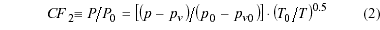The SAE J 1349 method indicates as a reference ambient condition for measuring the engine power in dynamometer tests p0 = 0.990 bar, T0 = 302.4 K, and p0n = 0.013 bar. This correction factor is recommended to be applied in the range from 0.950 to 1.010 bar, and from 288.5 to 316.3 K.

JIS D 1001 Method

The JIS D 1001 standard (JIS, 1993) recommends the following correction factor (CF3):The reference atmospheric condition for Eq. (3) is of p0= 1013.25 mbar and T0 = 293 K .

ISO 1585 Method

This method is given by ISO 1585 standard (ISO, 1992), and is also adopted by the Brazilian Society of Technical Standards (ABNT, 1996). The suggested power correction factor (CF4) is:The reference ambient condition is p0 = 1.000 bar, T0 = 298K and pn0 = 0.010 bar. The correction factor is recommended for utilization in the range from 0.800 to 1.000 bar, and from 288 to 308K.

Correlation between Acceleration Time and Engine Power

To evaluate the power developed by the vehicle engine from the measurements of acceleration time, the following data available from the vehicle manufacturer was considered in the calculation: mass distribution by shaft, drag coefficient, frontal area of vehicle, gear rate, engine curves of power and torque at a reference atmospheric condition, tire pressure for the conditions of the tests, tire circumference, coefficient of rolling resistance, mechanical efficiency, and friction factor for the road surface.

The power available to accelerate the vehicle is given as the difference between the output power from the engine and the road-load power, that is, the power necessary to overcome the rolling resistance which arises from the friction of the tires and the aerodynamic drag of the vehicle (Heywood, 1988):The engine output power was taken from the power curve available, corrected for the conditions of the road tests by the correction factors evaluated [Eqs. (1)-(5)]. From the vehicle speed, the rotational engine speed was calculated through Eq. (7):To evaluate the road-load power, the power to overcome the aerodynamic drag is first calculated: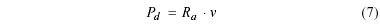andThus,The air density in Eqs. (8) and (9) is evaluated by the prefect gas law: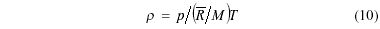Then, the power to overcome the rolling resistance is calculated (Gillespie, 1992):andThe road-load power is then given by: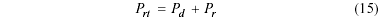The vehicle acceleration at a given speed v is given by:and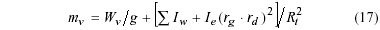The time interval Dt to reach a final speed nf from an initial speed ni is approached by: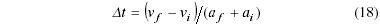To calculate the time interval Dt taken by the vehicle to reach a fixed distance (eg. 400 m, 1000 m), the following approach was adopted from the equation of motion:andThus, the time tf for the vehicle to reach a distance df, leaving from di at ti, ni and ai, is: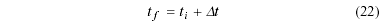and the vehicle speed nf at the time tfis written:Results

Figures 2 to 5 show the measured acceleration times and the acceleration times calculated through Eqs. 5 to 23 from the engine power output curve (Fig. 1) corrected by the correction factors CF1 to CF4. The engine power output curve (Fig. 1) used to determine the acceleration time was corrected to the road conditions from the laboratory ambient condition of 1 bar and 25 ° C. The experimental acceleration times shown are the average of ten tests performed at each atmospheric condition. Overall, Figs. 2 to 5 show that the acceleration time for a fixed distance or speed interval increases with increasing ambient temperature and decreases with increasing atmospheric pressure. These results were expected, as higher the air temperature as lower the air density and, therefore, the volumetric efficiency of the engine is diminished. On the other hand, higher atmospheric pressures help to increase the intake air mass charge, thus increasing the engine volumetric efficiency. Changing atmospheric pressure is seen from Figs. 2 to 5 to be more influential on the acceleration time than varying atmospheric temperature, for the ranges investigated.

Once the drag force is directly proportional to the air density, as shown by Eq. 8, an increase on air density through decreasing temperature or increasing pressure [Eq. (10)] while increasing the output power from the engine through a higher volumetric efficiency also augments the road-load power, diminishing the power available to accelerate the engine. In other words, higher pressures and lower temperatures would diminish the acceleration time for a fixed distance or speed interval through a higher power output, or provoke a different effect through a higher road-load power demand. The general trends observed from the results (Figs. 2 to 5) indicate that the effects of changing air density on the engine power output are stronger than on the road-load power.

The results show a higher slope for the calculated acceleration times in comparison to the experimental ones with varying temperature at 0.926-0.928 bar in the ranges from 40 to 100 km/h (Fig. 2), 0 to 400 m (Fig. 4) and 0 to 1000 m (Fig. 5). At this pressure level, the calculated acceleration times often over-predict the experimental acceleration times especially at the highest temperatures (29.4 to 33.8° C) of the range studied. At 1.026-1.027 bar the calculated acceleration times show a lower slope than the experimental acceleration times when the temperature is varied. Also, at this pressure level, the calculated values under-predict the experimental ones in most cases, especially in the ranges from 40 to 100 km/h (Fig. 2), 0 to 400 m (Fig. 4) and 0 to 1000 m (Fig. 5).

Figure 2 shows a comparison between the correction factors for the acceleration time from 40 to 100 km/h. The factors CF1, CF2 and CF3 furnish acceleration times calculated from the corrected engine standard power curve that are compared to the acceleration time measured in the experiments on the road. The acceleration time obtained from correction factor CF2 shows the lowest average discrepancy between road and laboratory experimental values, of 0.87%. The results from the correction factor CF1 are among the best, even though it does not consider air humidity in the calculation. The acceleration time calculated from the factor CF4 showed the highest average discrepancy from the road experimental values, of 2.0 %. Overall, the acceleration times obtained from the correction factors over-predict the values from the road experiments at the altitude of 827 m above sea level and under-predict the experimental values obtained at sea level for the temperature range investigated, with few exceptions. In general, correction factors gave acceleration times closer to the one measured by road experiments at the condition of 928 mbar and 22.8 ° C.

Figure 3 shows that, for the acceleration time from 80 to 120 km/h, the correction factor CF1 gives the results which best approach the road experimental values, with an average discrepancy of 1.31 %. The acceleration time from the correction factor CF2 shows the second best results, with an average discrepancy of 1.49 % from the road experiments. Correction factors CF4 and CF3 showed results which average discrepancies from the road experimental data were 1.59 and 2.34 %, respectively. In this speed range, all correction factors furnished acceleration times that over-predict the values from the road experiments at the altitude of 827 m above sea level, for all tested temperatures. At sea level, the correction factors gave acceleration times with a generally better approach to the road experimental data at the temperature of 28.2 ° C.

For the acceleration time from 0 to 400 m, the best results were again shown by correction factors CF1, CF2 and CF3, as Fig. 4 shows. The acceleration time calculated from correction factor CF2 showed the lowest discrepancy from the road experiments data, with an average value of 0.41 %. Correction factor CF4 showed the highest discrepancies from the road experimental values, of 0.81 %. The acceleration times calculated from the correction factors in this travel range showed the closest results to the road experimental data within the limits of pressures and temperatures tested.

The acceleration time for the largest range tested, from 0 to 1000 m, shows the results from correction factors CF1, CF2 and CF3 with a better approach to the road experimental results, as seen in Fig. 5. The acceleration time calculated from the correction factor CF2 showed the smallest average discrepancy from the road experimental data, of 0.33 %. The best approaches from the correction factor calculations were found for the atmospheric conditions at 827 m above sea level.

For most of the results obtained, correction factors CF1, CF2 and CF3 produced a better agreement between the laboratory and road experiments than correction factor CF4. Differently from the other three correction factors investigated, which consider a directly proportional relationship between the output power and the atmospheric pressure (Eqs. (1) to (3)), correction factor CF4 considers that the output power changes with atmospheric pressure to a power exponent of 1.2 (Eq. (4)). That is probably the reason why correction factor CF4 produced results with higher discrepancies.

From these results, one can conclude that the vehicle performance on the road has a different response to pressure, temperature and humidity variations than those proposed to correct engine power by Eqs. (1) to (4). The constants and considerations applied by Eqs. (5) to (23) to correlate the acceleration time and the engine power output may have influenced the differences observed between the calculated and experimental values. Direct measurement of the torque available in the wheels of the vehicle would eliminate the need to convert engine power output to acceleration time and, thus, provide a more precise evaluation of the power correction factors.

Conclusions

The atmospheric pressure was observed to be a more relevant parameter to influence the engine performance than the air temperature. Road tests confirmed that the acceleration time is increased with increasing air temperature and decreasing atmospheric pressure.

Trends observed in the results indicate that variations on the atmospheric temperature and pressure are more influential on the power output from the engine than on the road-load power.

The power correction factors evaluated in this work which showed the closest results to the road experimental data were the ones recommended by DIN 70020, SAE J 1349, and JIS D 1001 standards. From those, the correction factor indicated by SAE J 1349 standard is the one which produced the best results.

The vehicle acceleration time does not have a dependence on atmospheric pressure, temperature and humidity as described by the engine power correction factors evaluated in this work. Direct measurement of wheel torque on the road may allow for a better evaluation of the power correction factors.

Acknowledgment

The authors thank FIAT Automóveis S.A. for the financial support of this project.

• ABNT, 1996, "Road Vehicles  Engine Test Code  Net Effective Power" (In Portuguese), NBR/ISO 1585, Brazilian Association of Technical Standards.
• Chiu, C.P., and Horng, R.F., 1992, "Effects of Intake Air Temperature and Residual Gas Concentration on Cycle-to-Cycle Combustion Variation in a Two-Stroke Cycle S.I. Engine Equipped with an Air Assisted Fuel Injection System", JSME International Journal, Vol. 37, N.4, pp. 957-965.
• DIN, 1986, "Automotive Engineering; Maximum Speed; Acceleration and Other Terms; Definitions and Tests", DIN 70020, Deutsches Institut für Normung.
• Giacosa, D., 1986, "Motori Endotermici" (In Italian), 2 ed., Ulrico Hoelpi Editore S.p.A., Milan.
• Gillespie, T.D., 1992, "Fundamentals of Vehicle Dynamics", SAE, Inc., USA.
• Heywood, J.B., 1989, "Internal Combustion Engine Fundamentals", McGraw-Hill Book Co.
• ISO, 1992, "Road Vehicles  Engine Test Code  Net Power", ISO 1585, International Organization for Standardization.
• JIS, 1993, "Road Vehicles  Engine Power Test Code", JIS D 1001, Japanese Industrial Standard.
• Kline, S.J., and McClintock, F.A., 1953, "Describing Uncertainties in Single Sample Experiments", Mechanical Engineering, Vol. 75, pp. 3-8.
• Nakajima, K., Shinoda, K., and Onoda, K., 1969, "Experiments on Effects of Atmospheric Conditions on the Performance of an Automotive Gasoline Engine", SAE Transactions, SAE 690166, pp. 745-766.
• Pulkrabek, W.W., 1997, "Engineering Fundamentals of the Internal Combustion Engine", Prentice Hall, Inc.
• Redsell, M., Lucas, G.G., and Ashford, N.J., 1993, "Factors Affecting Car Fuel Consumption", Proc. Instn. Mechanical Engineers, Vol. 207, N. D8, pp. 895-904.
• SAE, 1995, "Engine Power Test Code  Spark Ignition and Compression Ignition  Net Power Rating", SAE J 1349, Society of Automotive Engineers.
• Soares, S.M.C., and Sodré, J.R., 2002, "Effects of Atmospheric Temperature and Pressure on the Performance of a Vehicle", Proceedings of the Institution of Mechanical Engineers Part D Journal of Automobile Engineering, Vol. 216, N. D6, pp. 473-477.
• Taylor, C.F., 1992, "The Internal Combustion Engine in Theory and Practice", The MIT Press, Vol. 1, Second Edition, Revised, USA, 574 p.
• Tsao, K.C., and Miller, E.M., 1984, "Performance of Gasoline-Water Fuel in a Modified SI Engine", SAE Technical Paper Series, SAE 841399, SAE, Inc.

# Publication Dates

• Publication in this collection
18 Mar 2004
• Date of issue
Sept 2003
Associação Brasileira de Engenharia e Ciências Mecânicas - ABCM Av. Rio Branco, 124 - 14. Andar, 20040-001 Rio de Janeiro RJ - Brazil, Tel.: +55 21 2221-0438, Fax: +55 21 2509-7129 - Rio de Janeiro - RJ - Brazil
E-mail: abcm@abcm.org.br